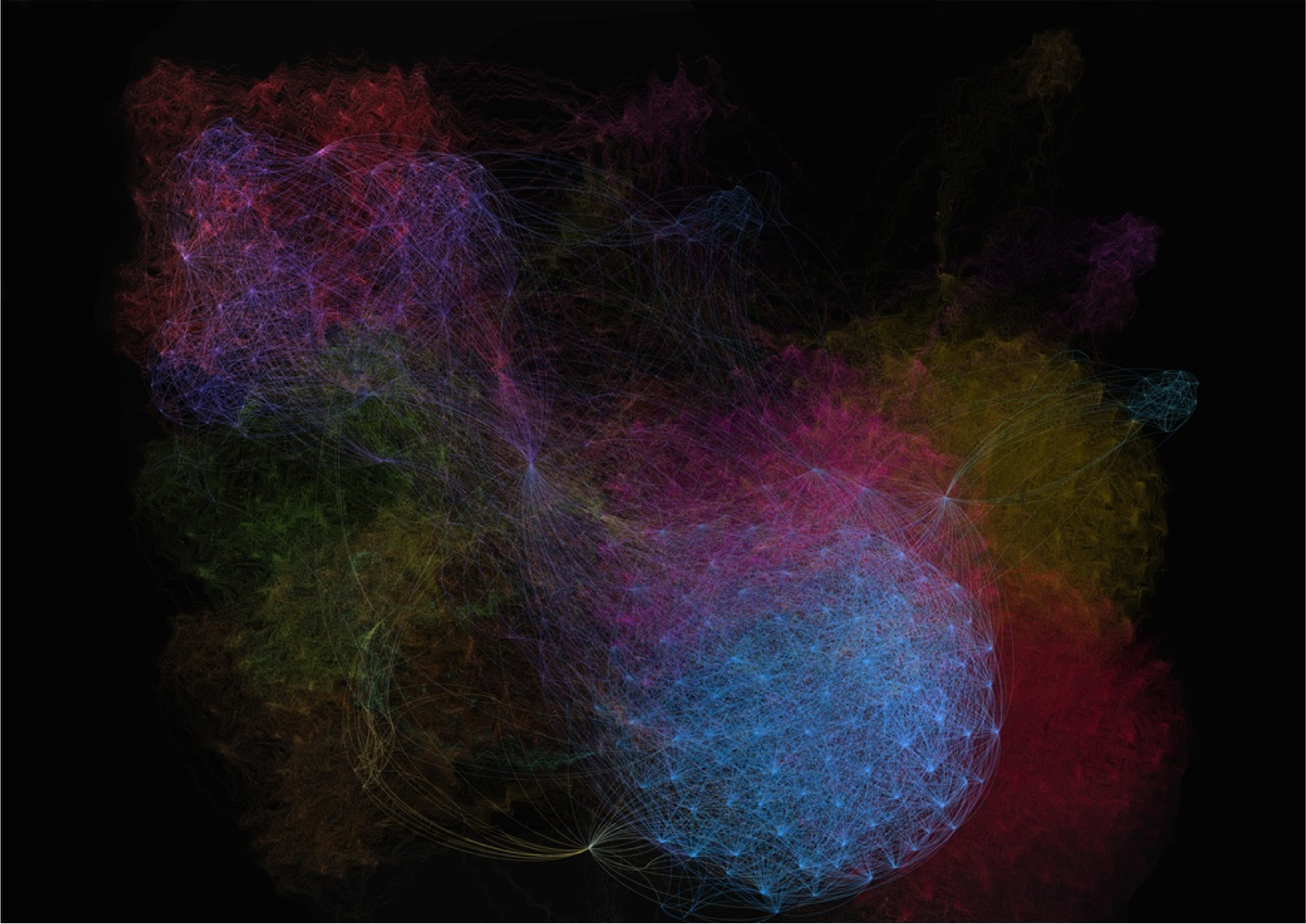# Graph Theory — History & Overview

### Part I — What Is Graph Theory & Why Is It Relevant Today?

November 27, 2018

Beyond CalculusGraphs, particularly network graphs, call my attention.

Maybe it’s the intuitive hunch that analyzing systems as graphs will grow my understanding of decentralized vs centralized networks. The mathematician in me sees how nailing down network analysis can greatly benefit research in incentive-driven systems. Or maybe it’s the designer in me largely attracted to the innate artistry of graphs like the one above — visually appealing, yet ultimately rich with information.

For whatever reason, after coming across graphs as trees in software development, as networks in blockchain research, or as r/dataisbeautiful click-bait, I decided to take a deep dive into the world of graph theory & it’s sub-branch of network theory.

The field of mathematics is large. It’s tree of knowledge branches into an ever-growing number of sub-fields. One of the highest level ways of subdividing & describing a set of branches is by the type of number within a given problem. Numbers in problems can either be discrete, as in fixed, terminable values such as natural numbers 1,2,3,4. Or they can be continuous, numbers that more accurately map our reality as dynamic, changing values like the rate of velocity of an object.

Discrete numbers are to an old-school digital clock what continuous numbers are to a modern analog clock (one that slides, not ticks). In the former, no numbers exist between 11:32 AM & the next minute 11:33 AM. In the latter, the second hand is continuously moving which means that the analog clock technically displays a different value every single moment as the second hand slides from one second to the next. The best example of a branch of math encompassing discrete numbers is combinatorics, the study of finite collections of objects. The best example of a branch of math based on continuous numbers is calculus, the study of how things change.

Graph theory, a discrete mathematics sub-branch, is at the highest level the study of connection between things. These things, are more formally referred to as vertices, vertexes or nodes, with the connections themselves referred to as edges.

# History of Graph Theory

The basic idea of graphs were first introduced in the 18th century by Swiss mathematician Leonhard Euler. His attempts & eventual solution to the famous Königsberg bridge problem depicted below are commonly quoted as origin of graph theory:

The German city of Königsberg (present-day Kaliningrad, Russia) is situated on the Pregolya river. The geographical layout is composed of four main bodies of land connected by a total of seven bridges. The question posed to Euler was straightforward: was it was possible to take a walk through the town in such a way as to cross over every bridge once, and only once (known as a Euler walk)?

Euler, recognizing that the relevant constraints were the four bodies of land & the seven bridges, drew out the first known visual representation of a modern graph. A modern graph, as seen in bottom-right image C, is represented by a set of points, known as vertices or nodes, that connected by a set of connecting lines known as edges.

By first attempting to draw paths in the graph above, then later experimenting with multiple theoretical graphs with alternating number of vertices & edges, he eventually extrapolated a general rule:

In order to be able to walk in an Euler path (aka without repeating an edge), a graph can have none or two odd number of nodes?

From there, the branch of math known as graph theory lay dormant for decades. In modern times, however, it’s application is finally exploding.

Applications of Graph Theory

Graph Theory is ultimately the study of relationships. Given a set of nodes & connections, which can abstract anything from city layouts to computer data, graph theory provides a helpful tool to quantify & simplify the many moving parts of dynamic systems. Studying graphs through a framework provides answers to many arrangement, networking, optimization, matching and operational problems.

As we move on to learning the basics of graph set & matrix notation (2), it can’t hurt to boost our autodidact motivation by covering a few applications — a peek of graph theory in action:

In software engineering, they’re known as a fairly common data structure aptly named decision trees. Over in the world of electrical engineering, an entire discipline revolves around the creation, calculation, & maintenance of multi-part electric circuits — often diagrammed following graph theory principles. Meanwhile in the realm of molecular biology, scientists extrapolate prediction models for tracking the spread of diseases or breeding patterns. And finally, in the controversial world of social media network analysis, we witness graph theory leveraged to create now-standard features such as LinkedIn’s degrees-of-separation & Facebook’s friend-recommendation features. Let’s move forward to the next article as familiarize ourselves with common graph notation.

sources

Introduction to Graph Theory

Graph Theory